# Drawing Geometric Primitives

The Java 2D API provides several classes that define common geometric objects such as points, lines, curves, and rectangles. These geometry classes are part of the `java.awt.geom` package.
The `PathIterator` interface defines methods for retrieving elements from a path.
The `Shape` interface provides a set of methods for describing and inspecting geometric path objects. This interface is implemented by the `GeneralPath` class and other geometry classes.

All examples represented in this section create geometries by using `java.awt.geom` package and then render them by using the `Graphics2D` class. To begin you obtain a `Graphics2D` object, for example by casting the `Graphics` parameter of the `paint()` method.

```public void paint (Graphics g) {
Graphics2D g2 = (Graphics2D) g;
...
}
```

## Point

The `Point` class creates a point representing a location in (x,y) coordinate space. The subclasses `Point2D.Float` and `Point2D.Double` provide correspondingly float and double precision for storing the coordinates of the point.

```//Create Point2D.Double
Point2D.Double point = new Point2D.Double(x, y);
```

To create a point with the coordinates 0,0 you use the default constructor, `Point2D.Double()`.
You can use the `setLocation` method to set the position of the point as follows:

• `setLocation(double x, double y)` – To set the location of the point- defining coordinates as double values.
• `setLocation(Point2D p)` – To set the location of the point using the coordinates of another point.

Also, the `Point2D` class has methods to calculate the distance between the current point and a point with given coordinates, or the distance between two points.

## Line

The `Line2D` class represents a line segment in (x, y) coordinate space. The `Line2D. Float` and `Line2D.Double` subclasses specify lines in float and double precision. For example:

```// draw Line2D.Double
g2.draw(new Line2D.Double(x1, y1, x2, y2));
```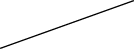This class includes several `setLine()` methods to define the endpoints of the line.
Aternatively, the endpoints of the line could be specified by using the constructor for the `Line2D.Float` class as follows:

• `Line2D.Float(float X1, float Y1, float X2, float Y2)`
• `Line2D.Float(Point2D p1, Point2D p2)`

Use the Stroke object in the `Graphics2D` class to define the stroke for the line path.

## Curves

The `java.awt.geom` package enables you to create a quadratic or cubic curve segment.

The `QuadCurve2D` class implements the `Shape` interface. This class represents a quadratic parametric curve segment in (x, y) coordinate space. The `QuadCurve2D.Float` and `QuadCurve2D.Double` subclasses specify a quadratic curve in float and double precision.

Several `setCurve` methods are used to specify two endpoints and a control point of the curve, whose coordinates can be defined directly, by the coordinates of other points and by using a given array.
A very useful method, `setCurve(QuadCurve2D)`, sets the quadratic curve with the same endpoints and the control point as a supplied curve. For example:

```// create new QuadCurve2D.Float
// draw QuadCurve2D.Float with set coordinates
q.setCurve(x1, y1, ctrlx, ctrly, x2, y2);
g2.draw(q);
```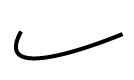### Cubic Curve Segment

The `CubicCurve2D` class also implements the `Shape` interface. This class represents a cubic parametric curve segment in (x, y) coordinate space. `CubicCurve2D.Float` and `CubicCurve2D.Double` subclasses specify a cubic curve in float and double precision.

The `CubicCurve2D` class has similar methods for setting the curve as the `QuadraticCurve2D`class, except with a second control point. For example:

```// create new CubicCurve2D.Double
CubicCurve2D c = new CubicCurve2D.Double();
// draw CubicCurve2D.Double with set coordinates
c.setCurve(x1, y1, ctrlx1,
ctrly1, ctrlx2, ctrly2, x2, y2);
g2.draw(c);
```## Rectangle

Classes that specify primitives represented in the following example extend the `RectangularShape` class, which implements the `Shape` interface and adds a few methods of its own.

These methods enables you to get information about a shape’s location and size, to examine the center point of a rectangle, and to set the bounds of the shape.

The `Rectangle2D` class represents a rectangle defined by a location (x, y) and dimension (w x h). The `Rectangle2D.Float` and `Rectangle2D.Double` subclasses specify a rectangle in float and double precision. For example:

```// draw Rectangle2D.Double
g2.draw(new Rectangle2D.Double(x, y,
rectwidth,
rectheight));
```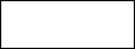The `RoundRectangle2D` class represents a rectangle with rounded corners defined by a location (x, y), a dimension (w x h), and the width and height of the corner arc. The `RoundRectangle2D.Float` and `RoundRectangle2D.Double` subclasses specify a round rectangle in float and double precision.

The rounded rectangle is specified with following parameters:

• Location
• Width
• Height
• Width of the corner arc
• Height of the corner arc

To set the location, size, and arcs of a `RoundRectangle2D` object, use the method `setRoundRect(double a, double y, double w, double h, double arcWidth, double arcHeight)`. For example:

```// draw RoundRectangle2D.Double
g2.draw(new RoundRectangle2D.Double(x, y,
rectwidth,
rectheight,
10, 10));
```## Ellipse

The `Ellipse2D` class represents an ellipse defined by a bounding rectangle. The `Ellipse2D.Float` and `Ellipse2D.Double` subclasses specify an ellipse in float and double precision.

Ellipse is fully defined by a location, a width and a height. For example:

```// draw Ellipse2D.Double
g2.draw(new Ellipse2D.Double(x, y,
rectwidth,
rectheight));
```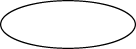## Arc

To draw a piece of an ellipse, you use the `Arc2D` class. This class represents an arc defined by a bounding rectangle, a start angle, an angular extent, and a closure type. The `Arc2D.Float` and `Arc2D.Double` subclasses specify an ellipse in float and double precision.

The `Arc2D` class defines the following three types of arcs, represented by corresponding constants in this class: OPEN, PIE and CHORD.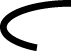Several methods set the size and parameters of the arc:

• Directly, by coordinates
• By supplied `Point2D` and `Dimension2D`
• By copying an existing `Arc2D`

Also, you can use the `setArcByCenter` method to specify an arc from a center point, given by its coordinates and a radius.

```// draw Arc2D.Double
g2.draw(new Arc2D.Double(x, y,
rectwidth,
rectheight,
90, 135,
Arc2D.OPEN));
```The `ShapesDemo2D.java` code example contains implementations of all described geometric primitives. For more information about classes and methods represented in this section, see the `java.awt.geom` specification.